# LLM Basics: Embedding Spaces—Transformer Token Vectors Are Not Points in Space

This post is written as an explanation of a misconception I had with transformer embedding when I was getting started. Thanks to Stephen Fowler for the discussion last August that made me realise the misconception, and others for helping me refine my explanation. Any mistakes are my own. Thanks to feedback by Stephen Fowler and JustisMills on this post.

TL;DR: While the token vectors are stored as n-dimensional vectors, thinking of them as points in vector space can be quite misleading. It is better to think of them as directions on a hypersphere, with a size component.

The I think of distance as the Euclidean distance, with formula:

Thus does not match up with the distance forumla used when calculating logits:

But it does match up with the cosine similarity forumula:

And so, we can see that the direction and size matter, but not the distance

# Introduction

In the study of transformers, it is often assumed that different tokens are embedded as points in a multi-dimensional space. While this concept is partially true, the space in which these tokens are embedded is not a traditional Euclidean space. This is because of the way probabilities of tokens are calculated, as well as how the behaviour of the softmax function affects how tokens are positioned in their space.

This post will have two parts. In the first part, I will briefly explain the relevant parts of the transformer, and in the second part, we will explore what is happening when a transformer moves from an input token to an output token explaining why tokens are better thought of as directions.

# Part 1: The Process of a Transformer

Here I will briefly describe how the relevant parts of the transformer work.

First, let’s briefly explain the relevant parts at the start of the transformer. We will be studying the “causal” transformer model (ie: that given tokens, we want to predict the ()th token). The main “pieces” of a causal model are:

• The Tokeniserturns “words” into “tokens” or “tokens” into “words”

• The Transformerturn N tokens into a prediction for the (N+1)th token

• Input Embedding—turns “tokens” into “vectors”

• Many Decoder layers—turns input vectors into prediction vectors

• with a Self-Attention sub-layer—uses information from all states

• with a Feed Forward sub-layer—uses information from the current state

• Output Unembedding—turns prediction vectors into token probabilities

Note that I won’t go into much depth about the positional and decoder layers in this post. If there is interest, I may write up another post explaining how they work if there is interest.

Also note, that for simplicity, I will initially assume that the input embedding and the output unembedding are the same. In this case, if you have a token, embed it, and then unembed it, you should get the same token out. The symmetry was true in the era of GPT-2, but nowadays, embedding and unembedding matrices are learned separately, so I will touch on some differences in the end.

Lastly, note that “unembedding” is actually a fake word, and usually it is just called the output embedding. I think unembedding makes more sense, so I will call it unembedding.

## 1. The Start of the Transformer Process

In the beginning of the transformer process, there is the Tokeniser (converting “words” into “tokens”) and the Token Embedding (converting “tokens” into “token vectors”/​”hidden-state vectors”):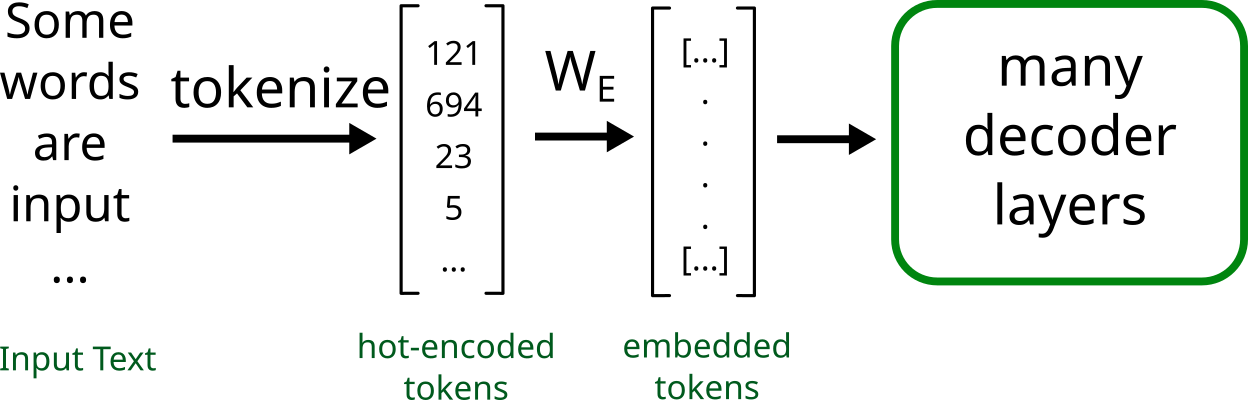So to start:

2. The text is split into different “parts” called token ids

3. The token ids are converted into vectors using the embedding matrix

4. The vectors are passed on into the rest of the transformer

### 1.1 The Tokeniser

The tokeniser is a separate piece of language model training which is used to try to make language more machine readable. It is usually trained once, separately to training the model. I will explain some intuition here, but this hugging face article explains tokenisers in more detail, if you are interested.

When we read a word like “lighthouse”, we don’t think if it in terms of the individual letters “l” “i” “g” “h” “t” “h” “o” “u” “s” “e”, but think of it as a whole word or concept. A subword tokeniser tries to compress the letters into meaningful words (eg: “lighthouse”) or parts of words (eg: “light” + “house”) using magical statistics to try to compress it as much as possible given a fixed limit for number of tokens.

The tokeniser, as usually defined, needs to be able to be able to compress all of english language into a “token” vocabulary of approx 50000. (usually up to 50400 so that it can run on TPUs or something, but I will just write 50000 since the exact number varies). The tokeniser does this compression by constructing a one-to-one map of token strings (combinations of characters) to token IDs (a number).

One common restriction, is that token strings are case-sensitive, so if you want to encode a word with the first letter capitalised, or written fully capitalised, these need to be separate tokens.

Another restriction, is that we usually want to be able to return the tokens back into text, and so need to be able to handle spaces and punctuation. For example, while we might be able to converts strings like “the cat” into”the” + “cat”, it is not certain how one should handle spaces and punctuation when converting it back from “the” + “cat” into “the cat”.

We could solve the spaces problem by adding a “space” token between each word, but this would make the input approx 2x longer, so is quite inefficient. We could always add a space between tokens, but this only works if all words are made up of one token, and are always separated by spaced. The solution in GPT-2, is to instead make the space a part of most tokens, by having a space padded before the word. That is, instead of “cat” we would write ” cat”.

Here is some example of the variations that arise for a common word like “next” due to the restrictions with case-sensitivity, as well as restrictions from padding a space:

We could also have tokens for punctuation like comma (“,”) or full stop (“.”) or spaces (” ”) for situations where they are needed, and in general, everything on the internet written in english should be able to be tokenised (though some things less efficiently than others).

Then, what the tokeniser does, is look at a string of text, split it into token strings, and turn that into a list of token IDs. This ie essentially a lookup table/​dictionary: For example:

• Start with “ The cat went up the stairs.”

• Separate out [ “ The”, “ cat”, “ went”, “ up”, “ the”, “ stairs”, “.” ]

• Store as “Token IDs”: tensor([[ 20, 4758, 439, 62, 5, 16745, 4]]

For less common tokens, the tokeniser usually has to split up the word into multiple parts that might not make as much sense from just looking at it. A somewhat common thing to happen, is have a “space+first letter” token, then a “rest-of-the-word” token. For example. ” Pickle” might become ” P” + “ickle”, but there are also other patterns.

While I gave some common restrictions above, you can have different restrictions, such as “make it so numbers are only tokenised by single digits”. In addition, there are usually some “special tokens”, such as “<|endoftext|>”x time. A useful way to get a better idea of tokenisation is the Redwood Research next-token prediction game.

### 1.2 The Input Embedding Matrix

The transformer, such as GPT-2 or Meta OPT, then uses an embedding matrix (WE) to convert its Tokens IDs into Token Vectors/​Hidden State Vectors. For the smallest transformer models, the hidden state vector size for is usually 768, and for larger models goes up to 4096. I will pretend that we are using a nice small model (mostly OPT-125m).

The size of the embedding matrix (WE) is (50000 * 768) for small models, where there are 50000 tokens and 768 dimensions. The unembedding matrix, which in our case computes the left inverse of the embedding matrix , is (768 * 50000) in size.

One way to think of the embedding matrix, is as a list of 50000 token vectors, one corresponding to each token, and then we use the token ID to take the right token vector. Here is a diagram showing the embedding of token IDs:Each token string gets a token ID, and these token IDs are turned embedded token vectors

The mathematical way we represent this conversion, is that we create a “token ID” vector that is the size of the token vocabulary, and make it 1.0 at the desired index, and 0 at all other indices. This makes it so that when we multiply the embedding matrix by this “token ID” vector, we get the vector for our token. This is just a different way choosing the vector from a list of vectors. The token ID is called a “hot-encoding” of the token ID vector, and corresponds to the index of the column in which the embedded token vector is stored. These extra steps are not important in the encoding, and are not physically done by machine (since it is essentially adding a lot of zeros), but are conceptually important for the decoding.

Here is an example for the token ” the” (which in the case of OPT-125m has token ID 5), which gives the same result as before: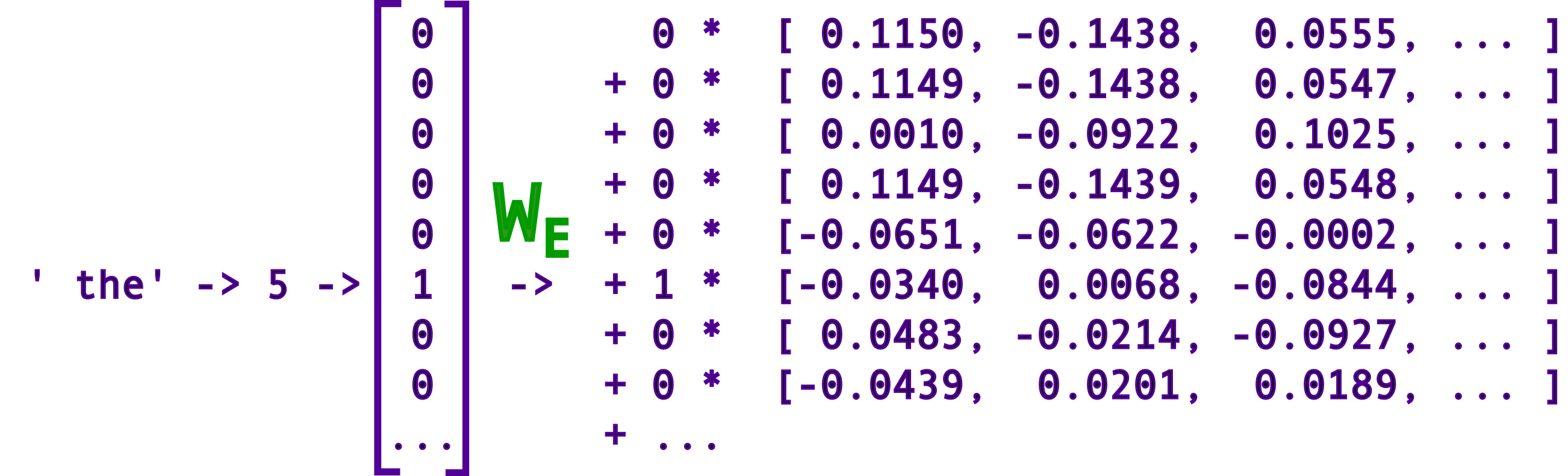The token ′ the’ gets converted into an ID, which hot-encodes a vector as shown, and is matrix multiplied and added up like above, such that we get the same result as before.

Here is an example of token embedding for the token ” NEXT” (which has token ID 10000)

Now, with a symmetric unembedding, if we wanted to get back the original token, we could then look to see which of the rows in the unembedding the vector is most similar to. We can look at what happens when we turn the token ID into its vector of zeros and ones, then embed it into a hidden state vector, and unembed that hidden state vector again straight away.

The input token ID vector is a nice clean vector with all zeros, except for at the Token ID we are encoding (in this case, token 10000, which is “ NEXT”). The output from the left inverse gives us the same value 1.0 at the index 10000, but due to the fact that we crammed 50000 tokens into a vector space of 768, we see some noisiness when trying to restore the original token, and see non-zero values at all the other Token ID Indices. The graph below shows what the result of an inverse unembedding might look like: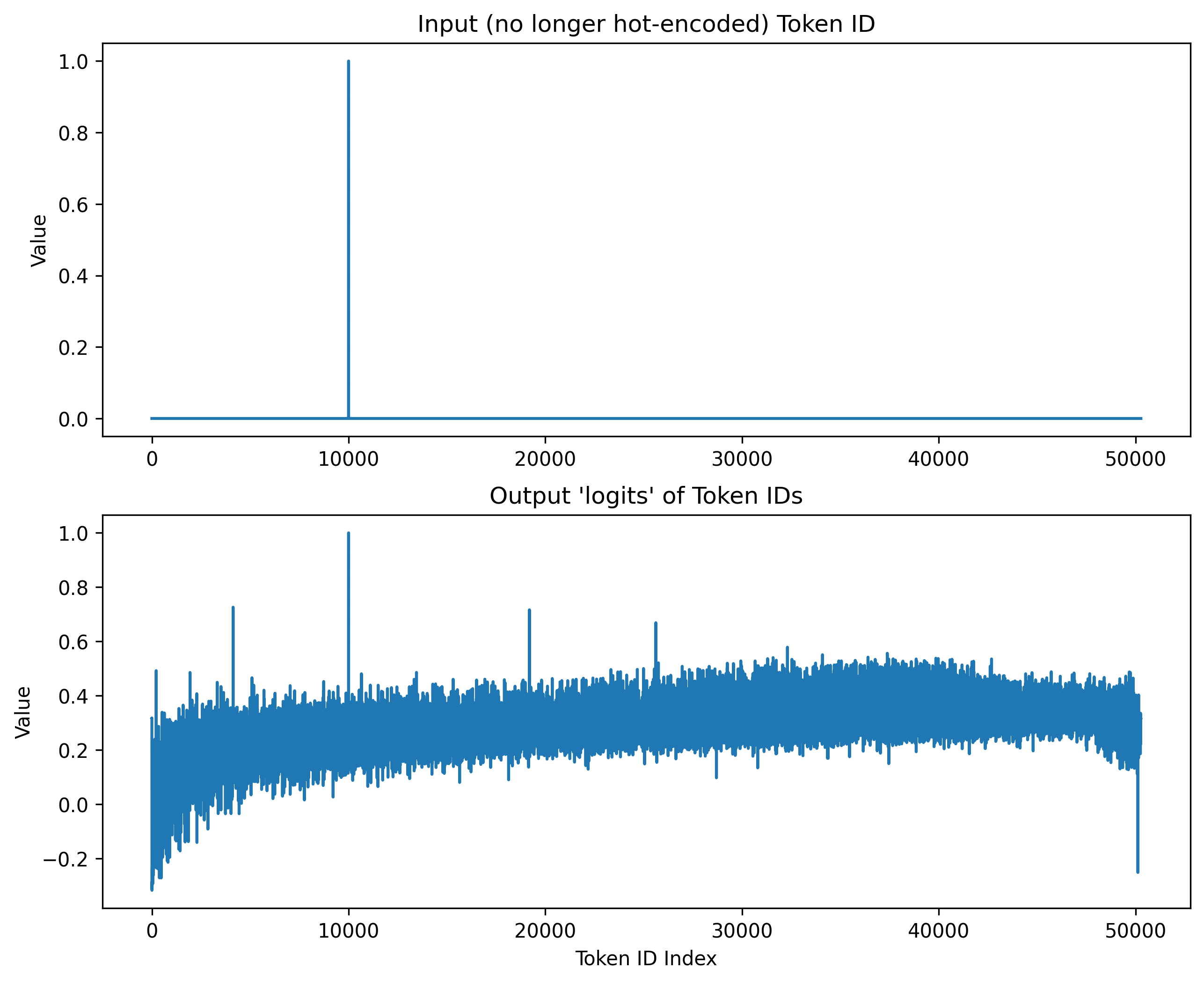Example of an input vector being embedded then unembedded in a (symmetric embedding) transformer, with an (artificially, by me) normalised inverse matrix

Most of the other high peaks in the diagram correspond to variations on the word “next” in different formats. This makes sense, since we expect them to be in a similar direction in vector space:

Note that in reality, instead of the inverse, they usually just use the transpose, and the pattern looks similar but slightly different, and we don’t get a nice peak of 1.0 at the value of the token we put in, but it is conceptually the same.

In some way, it is possible to think of space as having virtual points corresponding to each vector, and our real point vector starts off at one point corresponding to the vector that was input, and then after moving through the space, it finds a new point, which is the predicted output: the next token vector, decided by what the virtual tokens are. This is a useful picture, but lacks nuance, and it is better to think of the points more as “directions” in space instead of “points in space”, but I will describe the differnces in more detail after briefly describing the rest of the model.

### 1.3 Positional Encoding

In reality, I should also include positional encoding here, but it is not important for the discussion in this post, so I will skip it and bundle it into the “middle of the transformer process”.

## 2. The Middle of the Transformer Process

We will not care too much about what happens in the middle of the transformer. We will just assume that it magically knows how to get to the output token based on the previous inputs. Here is a diagram of a transformer decoder for reference:

Some summary of steps that do happen in a typical next-token-prediction model:

• The Hidden State Vectors get a “positional encoding” to keep track of the positions of the tokens (since the order of words and symbols in a text matters in English)

• The “Transformer Model” had many decoder layers which gradually make changes to the Hidden State Vectors, which should slowly become more “close” to the next predicted token

• The Self-Attention layers look at hidden states for all tokens and make adjustments to the current token based on that information

• The Feed-Forward Multi-Layer-Perceptron (MLP) Layers only look at the current hidden state and make adjustments based on that information

One key bit of information, is that in a typical model (though not necessarily all models), the hidden state vectors are normalised before being input to the Self-Attention, and the MLP. The layer norm means that all the transformer sees is the direction of the vectors.

## 3. The End of the Transformer Process

With the description of the parts before, we can now look at what happens at the output of the transformer model.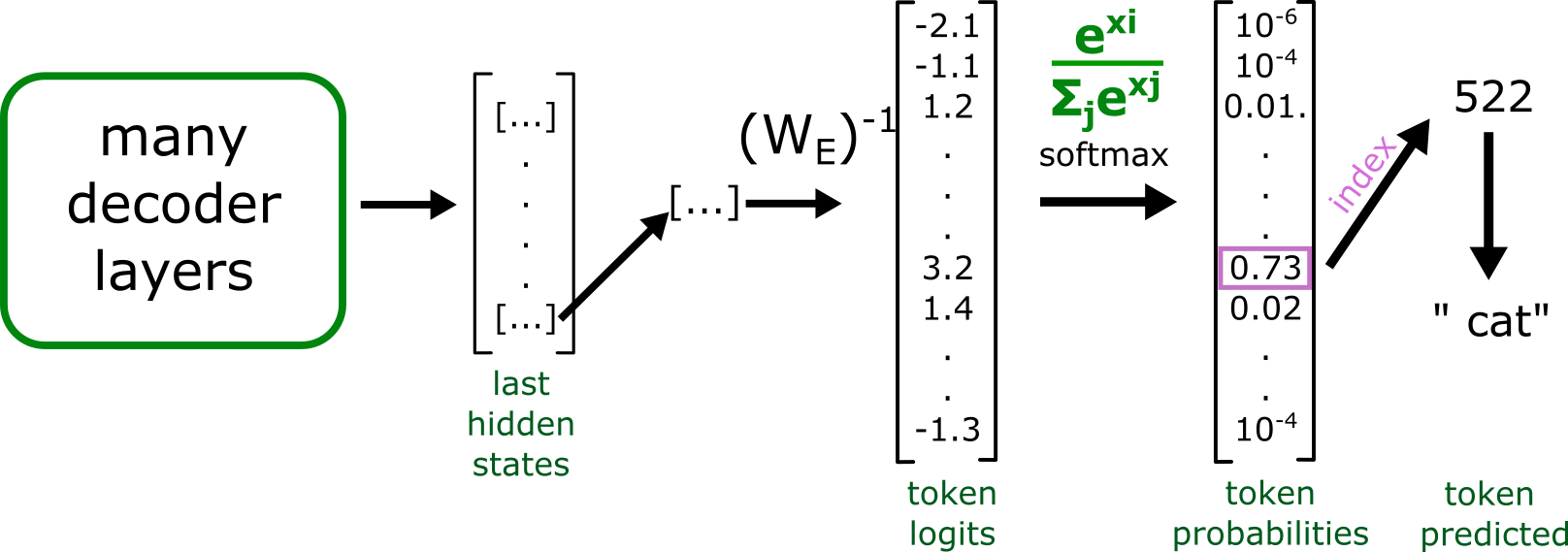Illustration of what happens at the end of a transformer run

Remember that we fed in a list of tokens into the transformer, and now we are trying to predict the th token.

The steps for next-token prediction in a transformer is then as follows:

1. In the output, we look at the final hidden state vector of the th token

2. The vector is multiplied by the unembedding matrix to get “logits,” (unnormalised log probabilities), for each possible token

3. The logits are softmax-ed to get a normalised probability distribution over the possible tokens

4. A token is chosen based on the probabilities

5. The token is converted back into text using the tokeniser dictionary

If we are running in a practical setting like ChatGPT, then we can then add the new ()th token to the input and run the model again to predict the ()th token, and this way predict a lot of extra text.

Side Note:

Since the model only looks at previous tokens (ie: not future tokens) when calculating the hidden states, it is possible to save the outputs from before and use a lot less compute than if we were running the whole model.

In addition, in training, what actually happens is that each of the [1, 2, .., N] input tokens give N output hidden states, and should predict tokens [2, 3, …, N+1] respectively. This means that in one run, you make N predictions instead of 1, which is why transformers are much easier to train than old recursive language models and scale much better.

### 3.1 Last hidden state vectors

As described before, for each token, we input a token hidden state vector, and get an output of a hidden state vector for that token. The optimiser is making it such that in the end, the model accurately predicts the next token.

### 3.2 The Output Unembed Matrix

We take the last hidden state vector, and multiply it by the unembed matrix. For symmetric models, it is simply given as the transpose:

If you wanted it to actually have the effect of being the inverse, and the tokens were not normalised, you would need to define it like below.

In practice, in symmetric models, they neither ensure normalisation, nor do they calculate the inverse like I have just shown. In more modern models, the unembed matrix is actually stored as a separate set of weights.

Then, the output is given by:

So what this formula means, is that the logit of each Token ID is just the dot product between the row of the unembed matrix () and the last hidden state vector (). So this formula for logits is how the “closeness to the output of a vector” is measured.

### 3.3 Softmax

Then, to get the actual probability of finding a token, we take the softmax, given by:

Where here is the “temperature”. For temperature 0, this is usually defined to be argmax (that is, the largest value is 1, and all others are 0), and for , this becomes a uniform distribution.

The best token then has a probability that can range from to 1.0. Here is an example of the “best token” probability with a range of temperatures: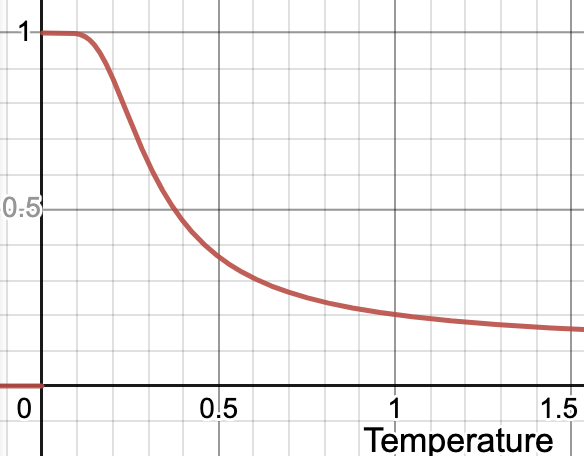Probability of best token at different temperatures, with 10 possible tokens. The fake token logits are [1.0, 0.01, 0.02, .. 0.09]

# Part 2: Misconception and Better Understanding

## “Points in Space” Explanation

Before thinking about it too much, I thought that the transformer:

• Encodes the initial vector that is the most recent word

• Pushes the hidden state vector towards the predicted word

• Chooses the token that is “closest” to that hidden state vector.

The process is true, but the word “closest” is misleading. In my mind, the word “closest” makes me think in terms of Euclidean distances. However, thinking in terms of Euclidean distances is is not an accurate way to think. In reality, the direction and size of the output vector matter more than its “position”. When extracting the logits, there is no Euclidean distance used, but instead, just unembedding with a matrix, as described above.

I was implicitly thinking that “distance” meant Euclidean distance, even though I knew there was no use of the euclidean distance formula:

Here is a flawed diagram of how I was thinking:

## “Directions in Space” Explanation

While the tokens are in fact encoded as vectors in a matrix, and can be drawn as points in n-dimensional space, it is much better to think only of the directions of the tokens, and not the actual points. So it is much better to think of them as points on an (n-1)-dimensional hypersphere with a size component. The real distance function is actually:

Which is a lot more similar to cosine similarity, which cares only about direction:

Here is a slightly less flawed diagram:

In addition, most tokens are roughly the same size, so it can be easier to think of the vectors as all roughly having the same size. We see below a diagram for the distribution of token vector sizes in the matrix for Meta’s OPT-125m model:OPT-125m distribution of embedded token vector sizes, on a log (left) and linear (right) scale. Most vectors are similar in size

We do see that there is a tail of tokens for which the tokens are quite different in size compared to the normal ones. In the case of OPT-125m, the unused tokens are very large, in other cases, the tokens can be very small. For an exploration on these weird tokens, I recommend “SolidGoldMagikarp (plus, prompt generation)” and “SolidGoldMagikarp II: technical details and more recent findings”

## The Effect of Vector Length

While the direction explains what the “closest tokens” are, how does the size affect the outcomes? As we know, the softmax gives a normalised distribution of probabilities, adding to a total of 1. The effect that having a larger vector is essentially the same as multiplying the logits by a multiple. The size difference makes it so that the logits are more spread out, and so, the outcome is that the model is “more confident” in a particular outcome.

Here is a diagram of how size makes a difference: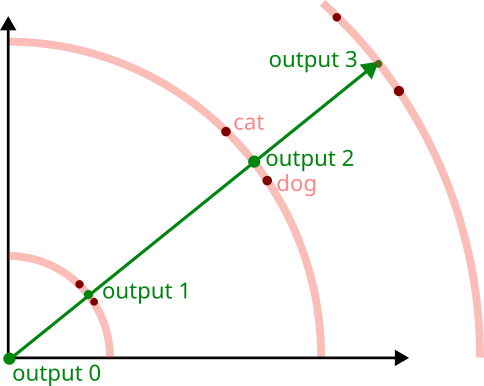At small lengths, difficult to differentiate directions. At large lengths, easy to differentiate directions.

In the limit of tokens being zero in size, the outcome is not pointing in any direction, so the probabilities of all tokens will be the same. With larger vectors, the model gets “more confident” in a particular outcome, as it is more able to distinguish the possible token directions. In essence, larger tokens ⇒ more “confidence”.

In addition, when the tokens are not quite the same length, we can sort of account for the size discrepancy by thinking of the directions on the hypersphere being a larger target. In theory, it is possible for some of the target tokens directions to overshadow other possible token directions. This seems to be happening in the “SolidGoldMagikarp (plus, prompt generation)” post I mentioned before.

The size only showing the difference in certainty is why it seems like the size of token vectors seems to grow to large vector sizes in the models I have checked. In a euclidean way of thinking, the growing sizes wouldn’t make sense, but with a direction-based thinking, it makes perfect sense. The plot below shows and example of vector norm size across layers for a few examples in OPT-125m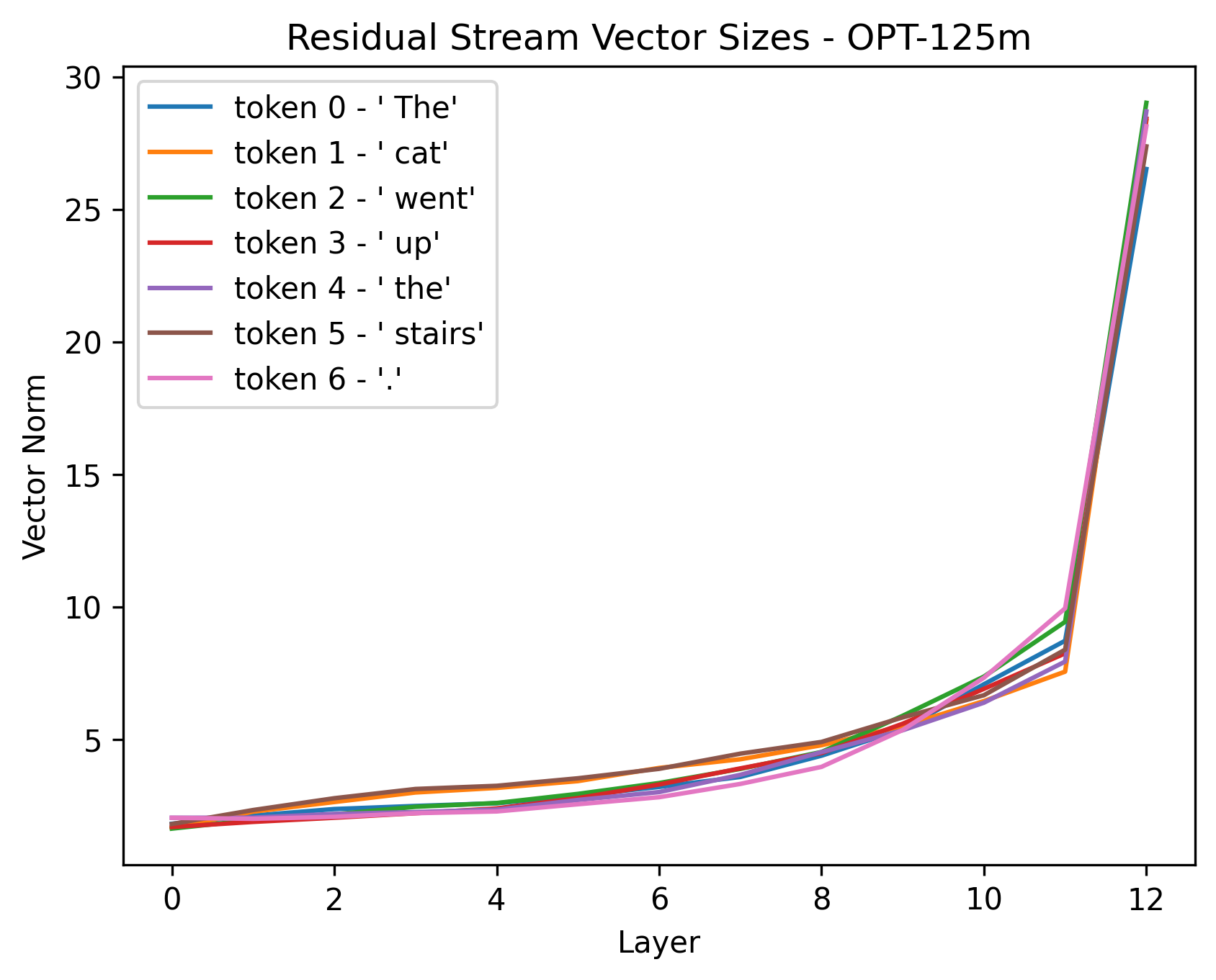How the size of hidden state vectors changes throughout different layers. At least in OPT-125M, for most tokens, it gets much larger.

So our final explanation for how the tokens change through space might look like the diagram below (somewhat cluttered due to excessive details):The hidden state direction is all that matters. In OPT-125m and some models, the length of the vector generally increases as it passes through mode layers

As a real example, we can look at a real model (OPT-125m) and look at the next-token prediction of:

“John and Mary went to the bar. John bought a drink for” → ” Mary”

(Example inspired by “Some Lessons Learned from Studying Indirect Object Identification in GPT-2 small). We see the same plot as before, but now in a real model.

The x and y coordinates are chosen to form a plane such that we can see the two tokens we are interested in. We see that there is some wandering in other dimensions for most of the start of the run initially (since I am only plotting 2 for the 768 dimensions), unit it finds out what it is supposed to be doing, and goes in the direction of the ” Mary” token.

Do note though that it is difficult to draw a 768 dimensional space, so while the 2d image might give some insights, do not take it too seriously.

# Non-symmetric Embeddings

While before I had been talking only about symmetric embeddings, in most modern models, symmetric embeddings are no longer used. Instead, the input and output embeddings are trained separately. The reasoning initially might have been that “it would make sense for the tokens to have a consistent token space”, but there are advantages to having a separate learned embedding.

One main thing, is that the requirement we have is not actually having a consistent token space, and being able to infer the input from the output. Instead, the task is next token prediction. Because of this, it makes sense for the output direction for likely next possible tokens to be in the same direction as the input direction. For example, in most contexts, a next word is not likely to be the previous word again, depending on the word, you can already do some bigram statistics on what the following word is.

For example: ” what” → ” the” or ” might” → ” be”, or ” How”, ” are”.

This separation of embedding spaces might be particularly useful for when token are split up into multiple parts, for example, something like ” Susp” → “icious”.

The key thing, is that the next predicted token is usually not the same as the input token, so it can be useful to have a separation.

The separation does mean, however, that when you are reasoning about what the vector directions are like in the middle of a transformer, that the hypersphere of directions may not correspond neatly to the input embedding space, or the output unembedding space, but might instead be something in between.

Previous research looking through the “logit lens” has mostly been looking at how the adjustments in the residual stream, and the activations in the residual stream, look from the point of view of the unembed matrix, so looking at the output logits seems more likely to be the more natural way of looking at things.

# Conclusion

While the tokens in transformers are stored as n-dimensional vectors, we should care not about the actual positions of the vectors in n-dimensional space, but instead, the directions of the vectors.

We can imagine the token vectors as directions on a (n-1)-hypersphere, with lengths showing how “large” the targets are on a hypersphere. The direction of the output decides what the next token should be, and the length decides the confidence.

1. ^

Feel free to look at how I generated some of the graphs in this python notebook.

• I’m pretty sure the main reason it’s good to think of features/​tokens as being directions is because of layer norm. All any “active” component of the neural network “sees” is the direction of the residual stream, and not its magnitude, since layer norm’s output is always norm 1 (before applying the scale and bias terms). You do mention this in part 1.2, but I think it should be mentioned elsewhere (e.g. Intro, Part 2, Conclusion), as it seems pretty important?

• I agree that the layer norm does some work here but I think some parts of the explanation can be attributed to the inductive bias of the cross-entropy loss. I have been playing around with small toy transformers without layer norm and they show roughly similar behavior as described in this post (I ran different experiments, so I’m not confident in this claim).

My intuition was roughly:
- the softmax doesn’t care about absolute size, only about the relative differences of the logits.
- thus, the network merely has to make the correct logits really big and the incorrect logits small
- to get the logits, you take the inner product of the activations and the unembedding. The more aligned the directions of the correct class with the corresponding unembedding weights are (i.e. the smaller their cosine similarity), the bigger the logits.
- Thus, direction matters more than distance.

Layernorm seems to even further reduce the effect of distance but I think the core inductive bias comes from the cross-entropy loss.

• I agree that there’s many reasons that directions do matter, but clearly distance would matter too in the softmax case!

Also, without layernorm, intermediate components of the network could “care more’ about the magnitude of the residual stream (whereas it only matters for the unembed here), while for networks w/​ layernorm the intermediate components literally do not have access to magnitude data!

• fair. You convinced me that the effect is more determined by layer-norm than cross-entropy.

• While I think this is important, and will probably edit the post, I think even in the unembedding, when getting the logits, the behaviour cares more about direction than distance.

When I think of distance, I implicitly think Euclidean distance:

But the actual “distance” used for calculating logits looks like this:

Which is a lot more similar to cosine similarity:

I think that because the metric is so similar to the cosine similarity, it makes more sense to think of size + directions instead of distances and points.

• Yeah, I agree! You 100% should not think about the unembed as looking for “the closest token”, as opposed to looking for the token with the largest dot product (= high cosine similarity + large size).

I suspect the piece would be helpful for people with similar confusions, though I think by default most people already think of features as directions (this is an incredible tacit assumption that’s made everywhere in mech interp work), especially since the embed/​unembed are linear functions.

• reasonable detail add. nitpick: points on a hypersphere are absolutely points in space.

• This is true. I think that visualising points on a (hyper-)sphere is fine, but it is difficult in practice to parametrise the points that way.

It is more that the vectors on the gpu look like , but the vectors in the model are treated more like

• Can you do a series on LLM basics?

• Do you know anything about the history of the unembedding matrix? Vaswani et al 2017 used a linear projection to the full size of the vocabulary and then a softmax to generate probabilities for each word. What papers proposed and developed the unembedding matrix? Do all modern models use it? Why is it better? Sources and partial answers would be great, no need to track down every answer. Great resource, thanks.

• I Googled up ‘how are tokens embedded’ and this post came up third in the results—thanks for the post!